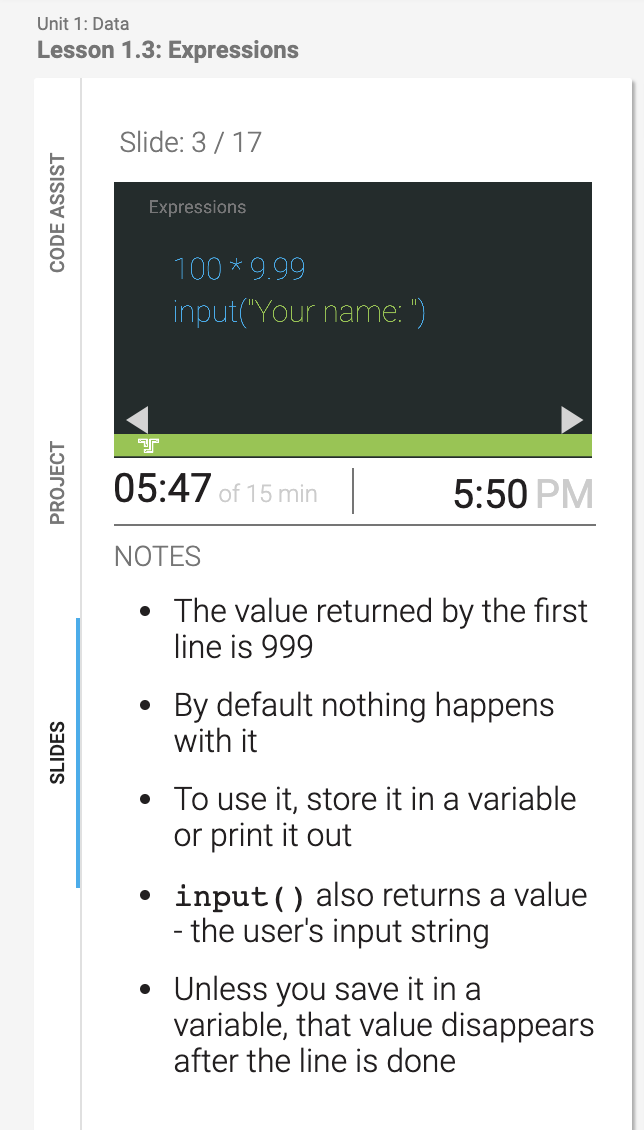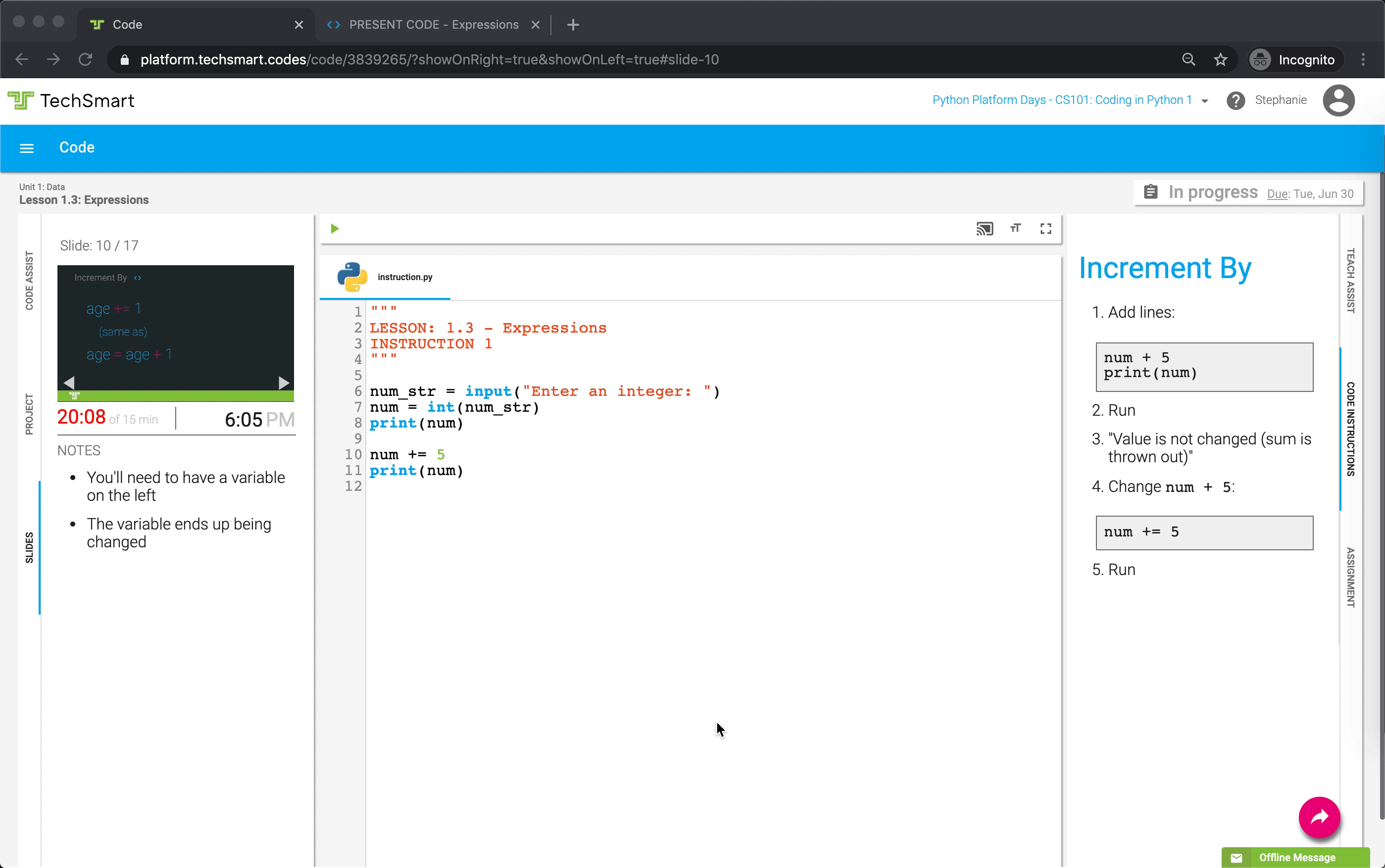# Instruction Activity Walkthrough

## Setting up an Instruction Activity

1. Go to the Lesson Plan Day View for the Instruction you are teaching - in this example, we're showing 1.3 Expressions.

2. Under Teaching Files, click the Code files dropdown menu& click on theClassroom Setup Button once it turns black, for the starter file you want to open.3. You should now have two different tabs open:

• Code  This is the tab you'll keep on your computer when teaching an activity.
• PRESENT SLIDES – This is the tab that will be projected to students, when you are in class.

4. Drag the PRESENT SLIDES tab to the projected screen where students are able to see it.

## Teaching an Instruction Activity

Types of Slides

### Title & Vocabulary Slide

Each Instruction Activity begins with a slide including the title of the lesson and any new vocabulary terms the lesson introduces.

#### Slide Flow

Introduce the Title and Vocabulary terms:

"In this lesson we'll be learning about Expressions. Our new vocabulary terms are Expression and Operator."

Read or summarize the Slide Notes:

• "We'll get values and combine them to make new ones"
• "We'll see how the computer can help us do some basic math"

### Vocabulary Term Slides

These slides introduce new Vocabulary Terms and include notes for explaining them to students. There are two or more Vocabulary Terms slides in each Instruction Activity, one slide for each new vocabulary term introduced.

#### Slide Flow

Introduce the Vocabulary Term and its definition, either by reading it, or having a student read it.

Summarize the Slide Notes:

•  "Expressions give us a value but don't do anything with it."
• "They're just a fragment of code that we need to do something with, they aren't a complete instruction like a statement."
• "Have you heard the word expression before?"
• "Can you come up with an example of an expression?"

### Code Example Slides

These slides are used to introduce new example code syntax. There is at least one Code Example slide in each Instruction Activity.#### Slide Flow

Introduce the Code Examples:

"Here are two examples of expressions."

Explain each example, using the Slide Notes:

• "The value returned by the first example is 999"
• "By default nothing will happen with that value."
• "To use it in our program, we have to either store it in a variable or print it out."
• "In the second example, the user is asked for input."
• "The input command also returns a value - the user's input string."
• "Unless you save the user's answer in a variable, the value they enter just disappears after the line is done."

### Coding Activity Slides

These slides share a code example with students, and provide instructions for you to walk students through trying out this example on their own computers. There is at least one Coding Activity slide in each Instruction Activity.

Note: Coding Activity Slides are marked by  a  <> symbol at the top#### Slide Flow

Introduce the examples:

"Here's an example of code that uses the Increment By operator. The code age += 1 increments age by 1, and it is the same as age = age + 1"

Summarize the Slide Notes:

• "When using the Increment By operator, the variable name must be on the left."
• "If this code was run, the value stored in the age variable would change."

<> Code Instructions:

After summarizing the Slide Notes, look to the CODE INSTRUCTIONS section in the right panel.

1. Click theicon to change the PRESENT tab to show <> Code2. Follow the instructions in the CODE INSTRUCTIONS to write the code for the Coding Activity.

Students should follow along and write code with you.3. Once you've completed the instructions, click theicon to change the PRESENT tab to show Slides again.

4. You can then advance to the next slide and continue, or, if this is the last slide, remind students to turn in their work by clicking the pink turn-in buttonin the bottom right corner.